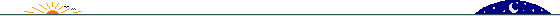Maris-McGwire-Sosa 7-tuples, 8-tuples, & 9-tuples

Mike Keith originated the concept of Maris-McGwire-Sosa k-tuples. Having looked for all MMS k-tuples (k>1) less than one billion, Mike noted two known 7-tuples: 1330820 [# 1] and 829885449 [# 2]. It was my intention to find more MMS 7-tuples, hoping (by chance) to luck into an example of an 8-tuple!

I am neither a very good programmer nor mathematician, so I come to rely on a high-level language (Mathematica) to do my dirty work. I build the program largely by trial and error, often looking up possibly useful functions and forcing them to carry out the algorithm as I understand it. Fortunately my sense of logic is relatively unimpaired and I can usually get programs to spew out appropriate results.

However, my approach is also an evolving one. After more than a year of using what I thought was a fairly optimized procedure, I realized that I did not need to factor every number as I had been doing. This occurred to me only after I started looking for larger MMS 7-tuples, where I knew factorization would be the bottleneck. As it turns out, if I had been using this improved algorithm all along, I would have been able to conduct my search in significantly less time.

At any rate, here is my list of MMS 7-tuples greater than one billion:

1011516574 [# 3]

1037580129 [# 4]

1049629254 [# 5]

1065469914 [# 6]

1680499518 [# 7]

2628845844 [# 8]

2967323193 [# 9]

2989325672 [#10]

3249880870, 3249880871, 3249880872 [27 November 1999: The first 9-tuple! See below.]

3251971448 [#11]

3475867810 [#12]

3864758410 [#13]

3920885902 [#14]

4041749790 [#15]

4042834880 [#16]

4274288185 [#17]

4398712766 [#18]

4799623343 [#19]

6209832453 [#20]

6353088369 [#21]

6372892664 [#22]

6405400704 [#23]

7314257525 [#24]

7864475024 [#25]

8083947851 [#26] Emily

8132540548 [#27] Emily

8378630642 [#28] Emily

8394260716 [#29] Emily

9346477539 [#30] Catherine

9761417745 [#31]

10039051880 [#32]

10116525677 [#33]

10968034765 [#34]

11176064522 [#35] Emily

11617191455 [#36] Emily

11631617516 [#37] Emily

12222533493, 12222533494 [13 December 2000: Finally, the first 8-tuple! See below.]

12536924737, 12536924738 [19 December 2000: Another one!]

12768529447 [#38]

12831752421 [#39]

13428282623 [#40] Catherine

13896306686 [#41]

14525284970, 14525284971 [6 January 2001]

14656636492 [#42]

14759783487 [#43]A Large MMS 7-tuple:

Since the very first 7-tuple appears shortly after 2^20, I thought it might be interesting to search for the first 7-tuple greater than 2^40. This particular result was achieved on a 400 MHz iMac DV:

*start* [2000/12/28, 19:16]

>2^20 [2000/12/28, 19:17] 1330820

>2^40 [2001/01/09, 10:41] 1100142936620The first 9-tuple:

3249880870 = 2 x 5 x 71 x 4577297 [49 + 56 = 105]

3249880871 = 17 x 53 x 409 x 8819 [50 + 55 = 105]

3249880872 = 2 x 2 x 2 x 3 x 7 x 421 x 45949 [51 + 54 = 105]

3249880873 = 193 x 16838761 [52 + 53 = 105]

3249880874 = 2 x 283 x 5741839 [53 + 52 = 105]

3249880875 = 3 x 3 x 5 x 5 x 5 x 43 x 67181 [54 + 51 = 105]

3249880876 = 2 x 2 x 11 x 73860929 [55 + 50 = 105]

3249880877 = 31 x 709 x 147863 [56 + 49 = 105]

3249880878 = 2 x 3 x 19 x 313 x 91079 [57 + 48 = 105]

The first 8-tuple:

12222533493 = 3 x 3 x 41 x 89 x 372173 [36 + 51 = 87]

12222533494 = 2 x 31 x 197137637 [37 + 50 = 87]

12222533495 = 5 x 43 x 107 x 531299 [38 + 49 = 87]

12222533496 = 2 x 2 x 2 x 3 x 317 x 1606537 [39 + 48 = 87]

12222533497 = 1543 x 1559 x 5081 [40 + 47 = 87]

12222533498 = 2 x 7 x 873038107 [41 + 46 = 87]

12222533499 = 3 x 11 x 1277 x 290039 [42 + 45 = 87]

12222533500 = 2 x 2 x 5 x 5 x 5 x 23 x 97 x 10957 [25 + 62 = 87]

This means, of course, that 12222533493 is the 7th term in A045760 and 3249880870, the 8th.9 January 2001 © Rarebit Dreams